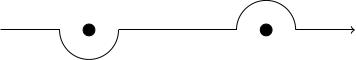# The Heisenberg picture and causality

## Schrödinger picture

In the Schrödinger picture of the Klein-Gordon field, the state of the system at any one time $$t$$ is described by a vector $$|\psi(t)\rangle$$ in a Hilbert space. As time varies, the state vector evolves according to the Schrödinger equation: $i\frac{d}{dt}|\psi(t)\rangle=H|\psi(t)\rangle,$ where $$H$$ is the quantum Hamiltonian operator and we are working in units where $$\hbar=1$$. The solution of this equation with initial state $$|\psi(0)\rangle$$ is $|\psi(t)\rangle=e^{-iHt}|\psi(0)\rangle,$ assuming the system is autonomous, that is the Hamiltonian has no time dependence.

## The Heisenberg picture

The Heisenberg picture emphasises instead the operators $$A$$ as dynamical objects which vary in time according to the Heisenberg equation $\frac{d}{dt}A(t)=i[H,A(t)].$ If $$H$$ is autonomous then a solution is given by $$A(t)=e^{iHt}A(0)e^{-iHt}$$. Note that if $$|\psi(t)\rangle$$ solves the Schrödinger equation and $$A(t)$$ solves the Heisenberg equation, then $\langle\psi(0)|A(t)|\psi(0)\rangle=\langle\psi(t)|A(0)|\psi(t)\rangle,$ so the two pictures are equivalent as far as computing expectation values is concerned.

## Our favourite operators in the Heisenberg picture

For the Klein-Gordon system, the creation and annihilation operators, $$a_\mathbf{p}^\dagger$$ and $$a_{\mathbf{p}}$$, satisfy the following commutation relations with the Hamiltonian \begin{align*} [H,a_{\mathbf{p}}]&=-\omega_{\mathbf{p}}a_{\mathbf{p}},\\ [H,a^{\dagger}_{\mathbf{p}}]&=\omega_{\mathbf{p}}a^{\dagger}_{\mathbf{p}}. \end{align*} The Heisenberg equation becomes \begin{align*} \frac{d}{dt}a_{\mathbf{p}}(t)&=-i\omega_{\mathbf{p}}a_{\mathbf{p}},\\ \frac{d}{dt}a^{\dagger}_{\mathbf{p}}(t)&=-i\omega_{\mathbf{p}}a^{\dagger}_{\mathbf{p}} \end{align*} and the solutions are \begin{align*} a_{\mathbf{p}}(t)&=e^{-i\omega_{mathbf{p}}}a_{\mathbf{p}}(0),\\ a^{\dagger}_{\mathbf{p}}(t)&=e^{-i\omega_{mathbf{p}}}a^{\dagger}_{\mathbf{p}}(0). \end{align*} The operators $$\phi(x)=\phi(\mathbf{x},t)$$ and $$\pi(x)=\pi(\mathbf{x},t)$$ are therefore given by \begin{align*} \phi(x)&=\int\frac{d^3 p}{(2\pi)^3}\frac{1}{\sqrt{2\omega_{\mathbf{p}}}}\left(a_{\mathbf{p}}(0)e^{-ip\cdot x}+a^{\dagger}_{\mathbf{p}}(0)e^{ip\cdot x}\right)\\ \pi(x)&=-i\int\frac{d^3 p}{(2\pi)^3}\sqrt{\frac{\omega_{\mathbf{p}}}{2}}\left(a_{\mathbf{p}}(0)e^{-ip\cdot x}-a^{\dagger}_{\mathbf{p}}(0)e^{ip\cdot x}\right) \end{align*} where $$p=(\omega_{\mathbf{p}},\mathbf{p})$$ and $$p\cdot x=\omega_{\mathbf{p}}t-\mathbf{p}\cdot\mathbf{x}$$.

## Causality

Recall that $[a_{\mathbf{p}},a^{\dagger}_{\mathbf{q}}]=(2\pi)^3\delta(\mathbf{p}-\mathbf{q}).$ Using this commutation relation, we have \begin{align*} [\phi(x),\phi(y)]&= \int\frac{d^3 p}{(2\pi)^3}\frac{1}{\sqrt{2\omega_{\mathbf{p}}}} \int\frac{d^3 q}{(2\pi)^3}\frac{1}{\sqrt{2\omega_{\mathbf{q}}}} \left([a_{\mathbf{p}},a^{\dagger}_{\mathbf{q}}]e^{iq\cdot y-p\cdot x} +[a^{\dagger}_{\mathbf{p}},a_{\mathbf{q}}]e^{ip\cdot x-q\cdot y}\right)\\ &=\int\frac{d^3 p}{(2\pi)^3}\frac{1}{2\omega_{\mathbf{p}}}\left(e^{ip\cdot(y-x)}-e^{ip\cdot(x-y)}\right)\\ &=D(x-y)-D(y-x), \end{align*} where we have defined $D(z):=\int\frac{d^3 p}{(2\pi)^3}\frac{1}{2\omega_{\mathbf{p}}}e^{-ip\cdot z}.$ The function $$D(z)$$ is Lorentz invariant (the integration measure $$d^3 p/2\omega_{\mathbf{p}}$$ is the Lorentz invariant measure on the mass shell and the integrand $$e^{-ip\cdot z}$$ is certainly Lorentz invariant). If $$x$$ and $$y$$ are spacelike separated ($$(x-y)^2<0$$), then, after a Lorentz transformation, we can assume that they lie on the spacelike slice $$t=0$$. In that case the integrals $$D(x-y)$$ and $$D(y-x)$$ are equal when we make the change of variables $$\mathbf{p}\to-\mathbf{p}$$, so, if $$(x-y)^2<0$$ we get $[\phi(x),\phi(y)]=0.$ The quantum Klein-Gordon field is therefore causal: local operators at spacelike separations commute.

Note that the amplitude $\langle 0|\phi(x)\phi(y)|0\rangle$ for the process "particle is created at $$y$$ and moves to $$x$$ where it is destroyed" is equal to $$D(x-y)$$. This is actually nonzero (exponentially decaying) outside the lightcone and oscillatory inside the lightcone. However, when $$(x-y)^2<0$$, this amplitude is cancelled by the process "particle is created at $$y$$ and moves to $$x$$ where it is destroyed", as the expectation value of the commutator $$[\phi(x),\phi(y)]$$ is zero.

## The propagator

Consider the operators $$\phi(x)$$ and $$\phi(y)$$.

• The time-ordered product $$T\phi(x)\phi(y)$$ is either $$\phi(x)\phi(y)$$ or $$\phi(y)\phi(x)$$ (if $$x^0>y^0$$ or $$y^0\leq x^0$$ respectively).
• The normal-ordered product $$:\phi(x)\phi(y):$$ is the result of expressing $$\phi(x)$$ and $$\phi(y)$$ in terms of creation and annihilation operators, multiplying them together, and then, in each monomial built out of creation and annihilation operators, blithely moving all the creation operators to the left.
• Let $$\Delta_F(x-y)=\begin{cases}D(y-x)&\mbox{ if }x^0>y^0,\\D(x-y)&\mbox{ if }y^0\geq x^0\end{cases}$$. This is called the Feynman propagator.

$T\phi(x)\phi(y)=:\phi(x)\phi(y):+\Delta_F(x-y).$
We have $\phi(x)=\phi^+(x)+\phi^-(x)$ where \begin{align*} \phi^+(x)&= \int\frac{d^3 p}{(2\pi)^3}\frac{1}{\sqrt{2\omega_{\mathbf{p}}}} a_{\mathbf{p}}e^{-ip\cdot x}\\ \phi^-(x)&= \int\frac{d^3 p}{(2\pi)^3}\frac{1}{\sqrt{2\omega_{\mathbf{p}}}} a^{\dagger}_{\mathbf{p}}e^{ip\cdot x}. \end{align*} If we assume that $$x^0>y^0$$ then \begin{align*} T\phi(x)\phi(y)&=\phi(x)\phi(y)\\ &=(\phi^+(x)+\phi^-(x))(\phi^+(y)+\phi^-(y))\\ &=\phi^+(x)\phi^+(y)+\phi^-(x)\phi^+(y) +\phi^+(x)\phi^-(y) +\phi^-(x)\phi^-(y). \end{align*} All of the terms here are normal-ordered except $$\phi^+(x)\phi^-(y)$$. We have $\phi^+(x)\phi^-(y)=[\phi^+(x),\phi^-(y)]+\phi^-(y)\phi^+(x).$ The term $$\phi^-(y)\phi^+(x)$$ is normal-ordered; moreover, we have \begin{align*} [\phi^+(x),\phi^-(y)]&= \int\frac{d^3 p}{(2\pi)^3}\frac{1}{\sqrt{2\omega_{\mathbf{p}}}} \int\frac{d^3 q}{(2\pi)^3}\frac{1}{\sqrt{2\omega_{\mathbf{q}}}} \left[a_{\mathbf{p}},a^{\dagger}_{\mathbf{q}}\right] e^{i(q\cdot y-p\cdot x)}\\ &=\int\frac{d^3 p}{(2\pi)^3}\frac{1}{\sqrt{2\omega_{\mathbf{p}}}} \int\frac{d^3 q}{(2\pi)^3}\frac{1}{\sqrt{2\omega_{\mathbf{q}}}} (2\pi)^3\delta(\mathbf{p}-\mathbf{q})\\ &=\int\frac{d^3 p}{(2\pi)^3}\frac{1}{2\omega_{\mathbf{p}}}e^{ip\cdot(y-x)}\\ &=D(y-x). \end{align*} A similar calculation when $$y^0\geq x^0$$ gives $$D(x-y)$$.

The Feynman propagator can be written as $\Delta_F(z)=\int\frac{d^3 p}{(2\pi)^3}\int_C\frac{dp^0}{2\pi}\frac{ie^{-ip\cdot z}}{p^2-m^2},$ where the $$p^0$$-integral is taken along the following contour $$C$$ in the complex $$p^0$$-plane:where the two dots indicate the poles $$p^0=\pm\omega_{\mathbf{p}}$$.
If $$x^0>y^0$$ then we can close the contour $$C$$ with a "semicircle at infinity" in the lower half $$p^0$$-plane, $$p^0\sim Re^{i\theta}$$ for large $$R$$, as the factor $$e^{-ip^0(x^0-y^0)}$$ in the integrand will tend to zero along this semicircle. The value of the line integral along $$C$$ is therefore equal to the sum of residues of the integrand in the region of the plane below $$C$$, in other words, the residue of the integrand at $$p^0=\omega_{\mathbf{p}}$$. This is $$1/2\omega_\mathbf{p}$$, giving $\int\frac{d^3 p}{(2\pi)^3}\int_C\frac{dp^0}{2\pi}\frac{ie^{-ip\cdot z}}{p^2-m^2}=D(y-x)$ when $$x^0>y^0$$. Similarly, if $$x^0\leq y^0$$ then we can close the contour in the upper half $$p^0$$-plane and we obtain $\int\frac{d^3 p}{(2\pi)^3}\int_C\frac{dp^0}{2\pi}\frac{ie^{-ip\cdot z}}{p^2-m^2}=D(x-y).$

The integral in the lemma is often written as $\Delta_F(z)=\int\frac{d^4 p}{(2\pi)^4}\frac{ie^{-ip\cdot z}}{p^2-m^2+i\epsilon},$ as the poles of this integrand are at $$p^0=\pm\sqrt{m^2+|\mathbf{p}|^2-i\epsilon}$$, which are slightly above/below the real axis. We may send $$\epsilon\to 0$$ and deform the real axis to the contour $$C$$ without crossing the poles to recover the original integral.

Comments, corrections and contributions are very welcome; please drop me an email at j.d.evans at lancaster.ac.uk if you have something to share.

CC-BY-SA 4.0 Jonny Evans.